Balbharati Maharashtra State Board Std 12 Commerce Statistics Part 1 Digest Pdf Chapter 8 Differential Equation and Applications Ex 8.6 Questions and Answers.

## Maharashtra State Board 12th Commerce Maths Solutions Chapter 8 Differential Equation and Applications Ex 8.6

Question 1.
In a certain culture of bacteria, the rate of increase is proportional to the number present. If it is found that the number doubles in 4 hours, find the number of times the bacteria are increased in 12 hours.
Solution:
Let x be the number of bacteria in the culture at time t.
Then the rate of increase is $$\frac{d x}{d t}$$ which is proportional to x.
∴ $$\frac{d x}{d t}$$ ∝ x
∴ $$\frac{d x}{d t}$$ = kx, where k is a constant
∴ $$\frac{d x}{x}$$ = k dt
On integrating, we get
∫$$\frac{d x}{x}$$ = k∫dt
∴ log x = kt + c
Initially, i.e. when t = 0, let x = x0
∴ log x0 = k × 0 + c
∴ c = log x0
∴ log x = kt + log x0
∴ log x – log x0 = kt
∴ log($$\frac{x}{x_{0}}$$) = kt ……(1)
Since the number doubles in 4 hours, i.e. when t = 4, x = 2x0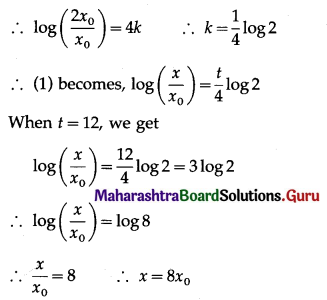∴ the number of bacteria will be 8 times the original number in 12 hours.Question 2.
If the population of a town increases at a rate proportional to the population at that time. If the population increases from 40 thousand to 60 thousand in 40 years, what will be the population in another 20 years? (Given: $$\sqrt{\frac{3}{2}}$$ = 1.2247)
Solution:
Let P be the population of the city at time t.
Then $$\frac{d P}{d t}$$, the rate of increase of population, is proportional to P.
∴ $$\frac{d P}{d t}$$ ∝ P
∴ $$\frac{d P}{d t}$$ = kP, k is a constant
∴ $$\frac{d P}{P}$$ = k dt
Integrating, we get
∫$$\frac{d P}{P}$$ = k∫dt
∴ log P = kt + c
Initially, i.e. when t = 0, P = 40000
∴ log 40000 = 0 + c
∴ c = log 40000
∴ log P = kt + log 40000
∴ log P – log 40000 = kt
∴ log($$\frac{P}{40000}$$) = kt ………(1)
When t = 40, P = 60000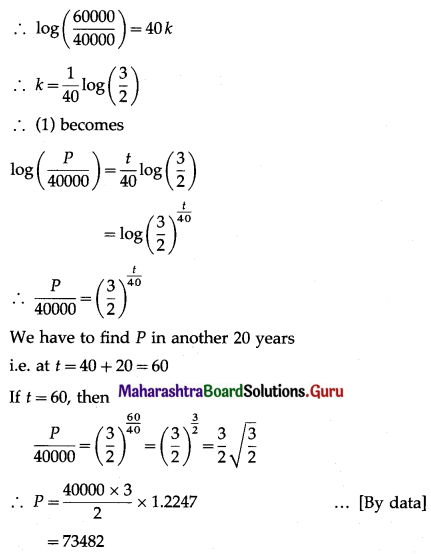∴ population after 60 years will be 73482.

Question 3.
The rate of growth of bacteria is proportional to the number present. If initially, there were 1000 bacteria and the number doubles in 1 hour, find the number of bacteria after $$\frac{5}{2}$$ hours. [Given: √2 = 1.414]
Solution:
Let x be the number of bacteria at time t.
Then the rate of increase is $$\frac{d x}{d t}$$ which is proportional to x.
∴ $$\frac{d x}{d t}$$ ∝ x
∴ $$\frac{d x}{d t}$$ = kx, where k is a constant
∴ $$\frac{d x}{x}$$ = k dt
On integrating, we get
∫$$\frac{d x}{x}$$ = k∫dt
∴ log x = kt + c
Initially, i.e. when t = 0, x = 1000
∴ log 1000 = k × 0 + c
∴ c = log 1000
∴ log x = kt + log 1000
∴ log x – log 1000 = kt
∴ log($$\frac{x}{1000}$$) = kt …….(1)
Now, when t = 1, x = 2 × 1000 = 2000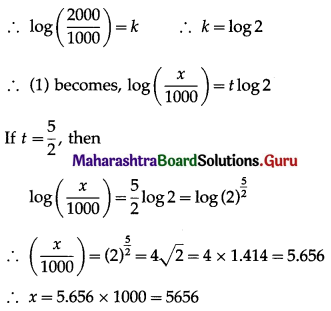∴ number of bacteria after $$\frac{5}{2}$$ hours = 5656.Question 4.
Find the population of a city at any time t, given that the rate of increase of population is proportional to the population at that instant and that in a period of 40 years, the population increased from 30,000 to 40,000.
Solution:
Let P be the population of the city at time t.
Then $$\frac{d P}{d t}$$, the rate of increase of population, is proportional to P.
∴ $$\frac{d P}{d t}$$ ∝ P
∴ $$\frac{d P}{d t}$$ = kP, where k is a constant.
∴ $$\frac{d P}{P}$$ = k dt
On integrating, we get
∫$$\frac{1}{P}$$dP = k∫dt
∴ log P = kt + c
Initially, i.e. when t = 0, P = 30000
∴ log 30000 = k x 0 + c
∴ c = log 30000
∴ log P = kt + log 30000
∴ log P – log 30000 = kt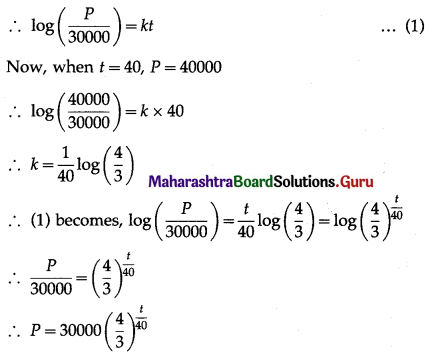∴ the population of the city at time t = 30000$$\left(\frac{4}{3}\right)^{\frac{t}{40}}$$.

Question 5.
The rate of depreciation $$\frac{d V}{d t}$$ of a machine is inversely proportional to the square of t + 1, where V is the value of the machine t years after it was purchased. The initial value of the machine was ₹ 8,00,000 and its value decreased ₹ 1,00,000 in the first year. Find the value after 6 years.
Solution:
Let V be the value of the machine at the end of t years.
Then $$\frac{d V}{d t}$$, the rate of depreciation, is inversly proportional to (t + 1)2.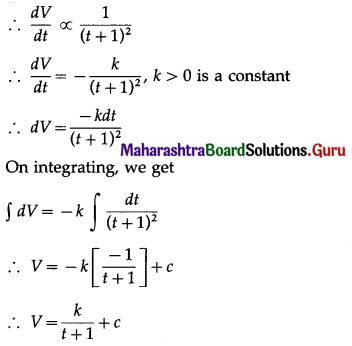Initially, i.e. when t = 0, V = 800000
∴ 800000 = $$\frac{k}{1}$$ + c = k + c ………(1)
Now, when t = 1, V = 800000 – 100000 = 700000
∴ 700000 = $$\frac{k}{1+1}$$ + c = $$\frac{k}{2}$$ + c ……(2)
Subtracting (2) from (1), we get
100000 = $$\frac{1k}{2}$$
∴ k = 200000
∴ from (1), 800000 = 200000 + c
∴ c = 600000 200000
∴ V = $$\frac{200000}{t+1}$$ + 600000
When t = 6,
V = $$\frac{200000}{7}$$ + 600000
= 28571.43 + 600000
= 628571.43 ~ 628571
Hence, the value of the machine after 6 years will be ₹ 6,28,571.